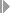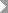Show TOC

###(01) Revenue-Based Method with Profit Realization#### Use

You can use the revenue-based method with profit realization for:

• Sales orders

• Projects

• Internal orders with revenue, service orders with revenue, maintenance orders with revenue

Revenue-based results analysis allows you to:

• Create reserves for unrealized costs

• Create reserves for imminent losses

• Use milestone billing

• Show interim profits

Profit realization is understood to mean showing interim profits. Interim profits arise when the revenue in a period is greater than the cost of sales and the difference is shown as a profit even though further revenues and costs are expected.

#### Prerequisites

You have planned costs and revenues.

Choose a results analysis method in simplified Customizing for Product Cost by Sales Order underPeriod-End ClosingResults AnalysisValuation Method.

#### Features

POC = R(a) / R(p)

C(PA) = COS * C(p) = R(a) / R(p) * C(p)

R(PA) = COS * R(p) = R(a) / R(p) * R(p) = R(a)

The revenue affecting net income equals the actual revenue. It can be allocated to CO-PA together with the cost of sales (costs affecting net income).

If the actual costs are greater than the costs affecting net income, the system creates capitalized costs (WIP).

If the actual costs are less than the costs affecting net income, the system creates reserves for unrealized costs.

Inventory values and reserves for unrealized costs can be transferred to FI and EC-PCA when you settle.

The cost of sales is zero as long as your actual revenue up to the results analysis period is zero. The capitalized costs then equal the actual costs incurred up to the results analysis period. Earnings are only calculated when revenues have been received.

#### Example

You have planned revenues of USD 200,000 and costs of USD 120,000 for your sales order.

Period 01

In period 01 you have actual costs of USD 20,000 but no revenues. In results analysis, the system calculates the following data:

• Capitalized costs in the amount of the actual costs (USD 20,000)

• Revenue of USD 0

• Cost of sales of USD 0

You then settle the capitalized costs to FI and EC-PCA. No line item is generated for CO-PA.

The following values are reported in CO-PA:

Profitability Analysis

 Revenues 0 Cost of sales 0 Result 0

The income statement shows the following values:

Income Statement

 Expense Revenue Actual costs 20,000 Inventory increase Capitalized costs 20,000 20,000 20,000

Period 02

In period 02 the actual costs increase by USD 60,000 and now total USD 80,000. You deliver to your customer and send him a milestone invoice for USD 100,000. The order is partially delivered and partially billed. In results analysis, the system calculates the following data:

• Cost of sales of USD 60,000 using the formula: Costs Affecting Net Income = Actual Revenue / Planned Revenue * Planned Costs

• Capitalized costs of USD 20,000 using the formula: Capitalized Costs = Actual Costs Costs Affecting Net Income if Actual Costs > Costs Affecting Net Income

• Revenue affecting net income of USD 100,000

You then settle the following:

• The cost of sales to CO-PA

• The revenues to CO-PA

• The change in the capitalized costs to FI and EC-PCA

The following values are reported in CO-PA:

Profitability Analysis

 Revenues 100,000 Cost of sales 60,000 Profit 40,000

The income statement shows the following values:

Income Statement

 Expense Revenue Actual costs 80,000 Actual revenue 100,000 Profit 40,000 Inventory increase Capitalized costs 20,000 120,000 120,000

If you are using the revenue-based method with profit realization, the system creates an income surplus in Financial Accounting of USD 40,000 that has not been realized on the market in the full amount. If you don’t want to report this surplus, you can use the revenue-based method without profit realization .

Period 03

In period 03 the actual costs increase to USD 90,000. You deliver a second amount to your customer and send him a second milestone billing for USD 90,000. The total revenue is now USD 190,000. The order is partially delivered and partially billed. In results analysis, the system calculates the following data:

• Cost of sales of USD 114,000 using the formula: Costs Affecting Net Income = Actual Revenue / Planned Revenue * Planned Costs

• Reserves for unrealized costs of USD 24,000 using the formula: Reserves for Unrealized Costs = Costs Affecting Net Income – Actual Costs if Actual Costs < Costs Affecting Net Income

The system cancels the capitalized costs of USD 20,000. The system creates reserves for unrealized costs in the amount of USD 24,000.

• Revenue affecting net income of USD 190,000

You then settle the following:

• The change in the cost of sales to CO-PA

• The change in the revenues to CO-PA

• The change in the capitalized costs and the reserves for unrealized costs to FI and EC-PCA

The following values are reported in CO-PA:

Profitability Analysis

 Revenues 190,000 Cost of sales 114,000 Profit 76,000

The income statement shows the following values:

Income Statement

 Expense Revenue Actual costs 90,000 Actual revenue 190,000 Reserves for unrealized costs 24,000 Profit 76,000 190,000 190,000

Period 04

In period 04 the actual costs increase to USD 130,000. You deliver the remaining goods and send the customer the final invoice for USD 10,000. The order is now fully delivered and fully invoiced.

In results analysis, the system calculates the following:

• Cost of sales of USD 130,000

Since the actual costs exceed the planned costs, the system sets the cost of sales to the value of the actual costs.

• No reserves for unrealized costs. The existing reserves are canceled.

• Revenue affecting net income of USD 200,000

You then settle the following:

• The change in the cost of sales to CO-PA

• The change in the revenues to CO-PA

• The cancellation of the reserves to FI and EC-PCA

The following values are reported in CO-PA:

Profitability Analysis

 Revenues 200,000 Cost of sales 130,000 Profit 70,000

The income statement shows the following values:

Income Statement

 Expense Revenue Actual costs 130,000 Actual revenue 200,000 Profit 70,000 200,000 200,000

The order has a total profit of USD 70,000.